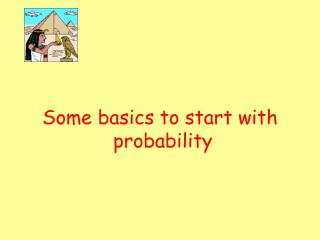Download PresentationDownload Presentation- - - - - - - - - - - - - - - - - - - - - - - - - - - E N D - - - - - - - - - - - - - - - - - - - - - - - - - - -
##### Presentation Transcript

2. Sample Space • A Sample Space is a LIST of all possible outcomes. • A Sample Space Diagram is a TABLE which shows all the possible outcomes of a scenario.

3. Independent Events Events are independent if the outcome of each event is not affected by any other event outcomes.

4. Example A bag contains 2 blue, 3 green and 5 red balls. If I draw one ball at random, the probability of a blue ball … P(Blue) = 2/10 If I draw another ball the probability of it being Blue depends on what colour ball was drawn first – not independent.

5. Probability - Notation The probability of an event A happening can be written as P(A) P(A) = number of ways event A can happen total number of possible outcomes (including A)

6. Example The probability of getting an “Ace” by drawing one card from a pack of 52 cards: P(Ace) = number of ways of getting an ace total number of possible cards picked = 4 52

7. Probability of Event NOT happening P(A’) is probability of event A NOT happening. P(A’) = 1 – P(A) e.g. P(not an ace) = 1 – 4 = 48 52 52

8. Probability of Independent Events If two events A and B are independent then the probability of them both happening is: P(A and B) = P(A) x P(B)

9. Example A dice and a coin are thrown. What is the probability of a HEAD and a 4? Answer: P(H and 4) = P(H) x P(4) = 1/2 x 1/6 = 1/12

10. Mutually Exclusive Events are mutually exclusive if they can’t happen at the same time e.g. If coin is tossed it cannot land as HEAD and TAIL Events “showing a head” or “showing a tail” are mutually exclusive.

11. Probability of Mutually Exclusive If two events A and B are mutually exclusive, the probability of either event A or event B occurring … P(A or B) = P(A) + P(B)

12. Example If a dice is thrown what is the probability of an even number or a “3” Since three is not an even number (!) P(Even or “3”) = P(Even) + P(3) = 3/6 + 1/6 = 4/6